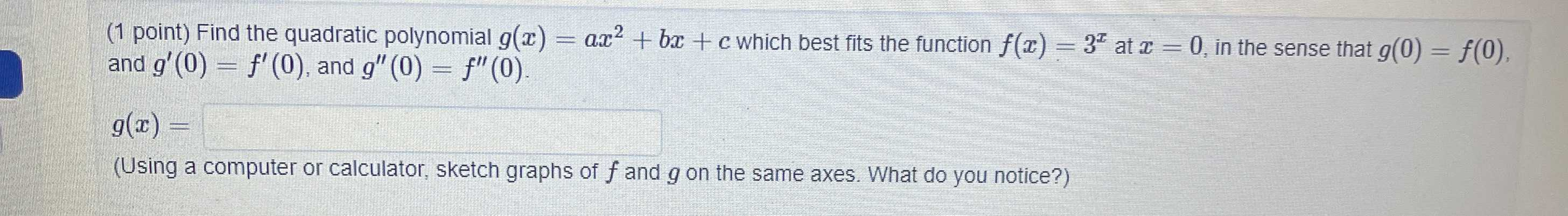### Still have math questions?Find the quadratic polynomial $$g ( x ) = a x ^ { 2 } + b x + c$$ which best fits the function $$f ( x ) = 3 ^ { x }$$ at $$x = 0$$ , in the sense that $$g ( 0 ) = f ( 0 )$$ , and $$g ^ { \prime } ( 0 ) = f ^ { \prime } ( 0 )$$ , and $$g ^ { \prime \prime } ( 0 ) = f ^ { \prime \prime } ( 0 )$$ . $$g ( x ) =$$ ___________.(Using a computer or calculator, sketch graphs of $$f$$ and $$g$$ on the same axes. What do you notice?)
$$g(0)= c= f(0)= 1\\g'(x)= 2ax+ b,f'(x)= 3^x\cdot\ln3\\f'(0)= \ln3= g'(0)= b\\g''(x)= 2a,f''(x)= 3^x\cdot(\ln3)^2\\g''(0)= 2a= f''(0)= (\ln3)^2\Rightarrow a= \frac{(\ln3)^2}{2}\\g(x)= \frac{(\ln3)^2}{2}x^2+ \ln3x+ 1$$